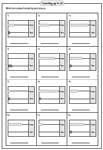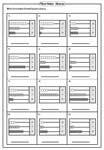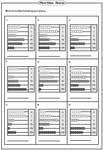Abacus worksheets in this page help students understanding place value of every digit starting from ones to hundred thousands. It also helps in learning composition and decomposition of numbers in base ten.

Each worksheet has 12 problems writing number shown by each abacus.Identify the 2-digit number shown by each abacus.Abacus worksheets in reading 3-digit numbers.Identify how many thousands, hundreds, tens and ones shown in each abacus.Each worksheet has 9 questions in reading 6-digit numbers.Level 1: Reading 2-digit, 3-digit, and 4-digit

Count the number of beads in each place value and find the number represented by abacus.Level 2: Reading 4-digit, 5-digit and 6-digit

This mixed review worksheets help to extend your knowledge in composing and decomposing hundred thousands, ten thousands and thousands.The entire collection of worksheets on abacus can be downloaded in a jiffy!

Related Worksheets